# AC Inductor Circuits

Want create site? Find Free WordPress Themes and plugins.

In an ac circuit, the applied voltage constantly varies and reverses polarity. Any inductance in the circuit will generate a counter emf, which will oppose the source voltage.

This event can be observed by setting up the experiment. Connect a light to a six- volt dc source. Note its brilliance. Now, connect the light to a six-volt ac transformer, and note the brilliance. Is it brighter or dimmer? The inductance has some indirect effect on the current.

Opposition to an alternating current due to inductance or capacitance is called reactance. The letter symbol for reactance is X. When reactance is caused by an inductor, it is called inductive reactance. Inductive reactance is measured in ohms, and its symbol is XL.

Induced Current and Voltage

Remember that the induced voltage in a coil is the counter emf. Counter emf opposes the source. Therefore, it is 180 degrees out of phase with the source voltage. This counter emf opposes change, and the greatest counter emf is induced when the current change is at a maximum.

Study Figure 1. The greatest rate of change of current is at 90 degrees and 270 degrees. At these points, the applied voltage must be at a maximum. The induced voltage is also at a maximum.

At 180 degrees and 360 degrees, the current change is minimal. The current is at its maximum value, ready to start its decline. As you can see in Figure 1, in a circuit containing pure inductor, the current is 90 degrees out of phase with the applied voltage. The current is lagging behind the voltage.Figure 1. Comparison of emf, counter emf, and current. The greatest counter emf occurs when the current is changing at its most rapid rate.

## Inductive Reactance or Inductor Impedance

The magnitude of the reactive force opposing the flow of ac is measured in ohms. It can be expressed mathematically as:

${{X}_{L}}=2\pi fL$

Where XL equals inductive reactance (inductor Impedance) in ohms, f equals frequency in hertz, L equals inductance in henrys, and π is approximately 3.1416. Remember, as frequency or inductance is increased, the inductive reactance increases. They are in direct proportion.

An inductance in a circuit behaves differently as various frequency signals are applied, Figure 2. An eight-henry inductor connected to a dc (f = 0) signal has a reactance of zero. This means the eight-henry inductor does not impede the dc signal at all.

Using the formula, XL = 2πfL, the reactance can be computed for frequencies of 50, 100, 500, and 1000 Hz. A partial graph of the results shows the linear increase of reactance as the frequency is increased.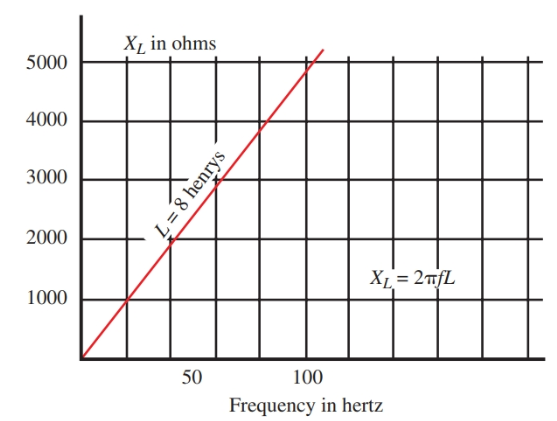Figure 2. As frequency increases, inductive reactance increases.

In Figure 3, the frequency has been held constant at 1000 Hz. The reactance is plotted as the inductance is increased from 0 henrys to 0.8 henrys. Notice the linear increase in reactance as the inductance is increased.

These principles can be applied to filter and coupling circuits. However, you must first understand the traits of reactance and how they change as a result of inductance and frequency of the applied voltage.Figure 3. As inductance increases, inductive reactance also increases.

## Power in Inductive Circuits

True Power: Power consumed in a purely resistive circuit is the product of the voltage and the current: P = I × E. This is the actual power used by a circuit. It is called true power, and it is given in units of watts.

Reactive Power: However, in an ac circuit containing inductance only, this formula does not hold true. In this type of circuit, a current will flow bound by the reactance of the circuit. The power used to build the magnetic field is returned to the source when the field collapses. This is reactive power. Reactive power is measured in VAR, which stands for volt-ampere-reactive.

Apparent Power: The combination of true power and reactive power gives rise to a measure of power that appears to be delivered to a load. This power is called apparent power. It is equal to the product of the effective voltage and the effective current. Apparent power is measured in VA or volt-amperes.

$ApparentPower={{E}_{ff}}\times {{I}_{ff}}$

## Apparent Power Calculation Example

A 100 Eeff is applied voltage to a certain inductive circuit. This causes an Ieff of 10 amps. The apparent power equals:

Apparent power = 100 V × 10 A = 1000 VA Remember, watts are not used for apparent power.

## Power Factor Definition & Formula

The ratio of true power to apparent power in an ac circuit is called the power factor (PF). It has no units. It can be calculated using trigonometry.

The power factor is equal to the cosine of the phase displacement between current and voltage. The formula is written:

$Power\text{ }Factor=\cos \theta =\frac{True\text{ }Power}{Apparent\text{ }Power}$

Where θ is the phase displacement. Note that by rearranging this formula, we arrive at the formula for true power.

\begin{align} & True\text{ }Power=Apparent\text{ }Power\times \cos \theta \\ & True\text{ }Power={{E}_{ff}}\times {{I}_{ff}}\times \cos \theta \\\end{align}

As an example, assuming our previous circuit is purely inductive, determine the power factor and phase displacement.

$PF=\cos \theta =\frac{0W}{1000VA}=0$

The power factor is 0. The angle whose cosine is 0 is 90 degrees. This tells us that current and voltage in the purely inductive circuit are 90 degrees out of phase, Figure 4.Figure 4. A circuit containing inductance only. The true power is zero and current lags the voltage by 90 degrees.

## Resistance and Inductance in an AC Circuit

If an ac circuit contains only resistance, the current and voltage are in phase, Figure 5. The power consumed is equal to I × E.

Even though the polarity of the voltage changes and the current reverses, positive power is consumed. A resistor consumes the same amount of power no matter which direction the current is moving. The power factor in a circuit of this type equals one. The apparent power is equal to the true power.

The circuit traits change when an inductor is added in series with the resistor. The component does not have to be a resistive component. The wire from which the coil is wound will have a certain amount of resistance.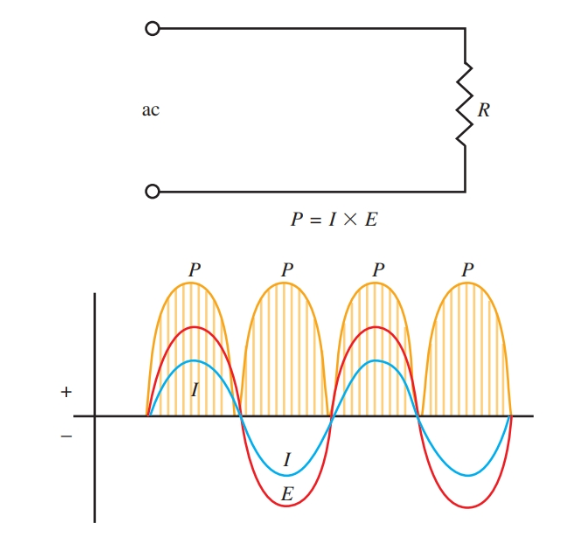Figure 5. In a purely resistive circuit, true power and apparent power are the same. The current and voltage are in phase.

Refer to Figure 6. The series resistance equals 300 ohms. The inductive reactance equals 400 ohms. This reactive component will cause the current to lag by an angle of 90 degrees or less. The forces opposing the current can be thought of as the resistance and reactance.

The resistance and reactance are 90 degrees out of phase. To find the final opposition, the combination of resistance and reactance, add the vectors of the two forces. Refer to Figure 7.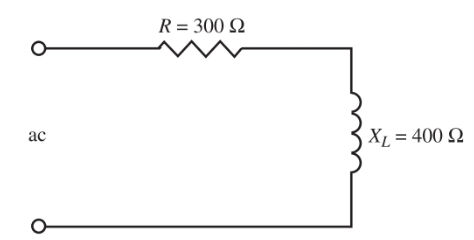Figure 6. RL circuit having a series resistance of 300 ohms and inductive reactance of 400 ohms.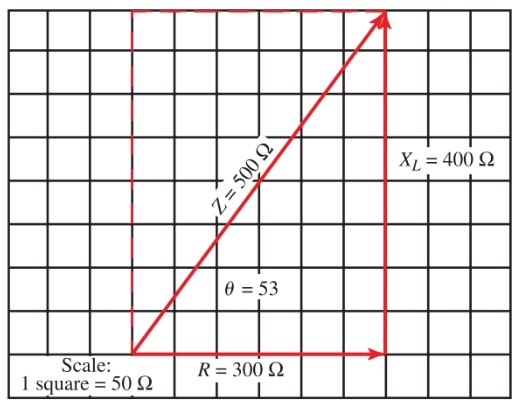Figure 7. Vector addition of XL and R, which are 90 degrees out of phase.

The vectors can be added using the graph. Place the tail of the XL vector on the arrowhead of the R vector. Then draw a vector from the starting point of the resistance vector to the head of the reactance vector, XL. This new vector shows the magnitude and direction of the combined forces. The angle between this new vector and the resistance vector is θ, see Figure 7.

## Impedance Definition & Formula

The total opposition to an alternating current in a circuit having resistance and reactance (the new vector you have just created) is the impedance. The symbol for impedance is Z, and it is measured in ohms.

Impedance problems are commonly solved using the Pythagorean Theorem. The Pythagorean Theorem states that the hypotenuse of a right triangle is equal to the square root of the sum of the squares of the two sides. It is commonly written:

$c=\sqrt{{{a}^{2}}+{{b}^{2}}}$

Where c is the hypotenuse and a and b are the other two sides of the right triangle.

In the problem of Figures 6 and 7, XL equals 400 and R equals 300. Find the impedance, Z.

$Z=\sqrt{{{R}^{2}}+X_{L}^{2}}=\sqrt{{{300}^{2}}+{{400}^{2}}}=500\Omega$

The impedance of the circuit is 500 ohms. The angle between vector Z and vector R is called the phase angle (θ). It represents the phase displacement between the current and voltage resulting from the reactive component. So, since cosθ equals the power factor:

$\cos \theta =\frac{R}{Z}=\frac{300}{500}=0.6$

Since the cosine of θ equals 0.6, the angle whose cosine is 0.6 (53.1° approximately) is equal to the phase angle. Therefore, the current lags the voltage by an angle of 53.1 degrees.

The true power in this circuit can now be calculated. The true power in this circuit equals the apparent power times the power factor, or cosθ.

\begin{align} & True\text{ }Power=Apparent\text{ }Power\times cos\theta \\ & \cos \theta =\frac{True\text{ }Power}{Apparent\text{ }Power} \\\end{align}

The waveforms for current, voltage, and power are drawn in Figure 8. Assuming an applied ac voltage of 100 V, the current in the circuit will equal:

$I=\frac{E}{Z}=\frac{100V}{500\Omega }=0.2A$

The apparent power equals:

$Apparent\text{ }Power=E\times I=100\times 0.2A=20VA$

The true power equals:

$True\text{ }Power=E\times I\times \cos \theta =100V\times 0.2A\times .6=12W$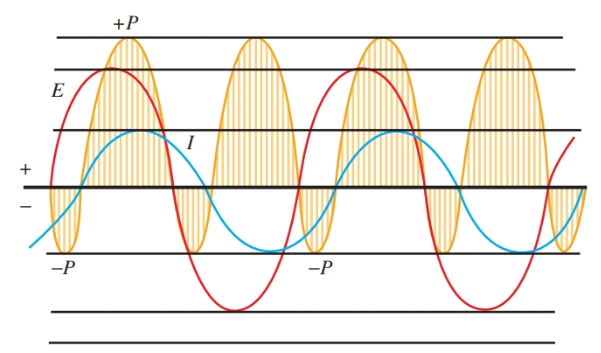Figure 8. The relationship between voltage, current, and power in the circuit described in the text.

These figures can be checked by inserting them into the power factor formula.

$PF=\cos \theta =\frac{True\text{ }Power}{Apparent\text{ }Power}=\frac{12W}{20VA}=0.6$

The power factor is not only considered in theory but in practice. The power factor must be considered whenever a power company connects power lines to a manufacturing plant. Industries must keep the power factor of their circuits and machinery within specified limits, or pay the power company a premium.

A reactive power is sometimes called wattles power. It is returned to the circuit. In Figure 8, the power in the shaded areas above the zero line is used. The power below the line is wattles power.

## Ohm’s Law for AC Circuits

In computing circuit values in ac circuits, Ohm’s law is used with one exception. Z is used in place of R. Z represents the total resistive force opposing the current. Therefore:

$\begin{matrix} I=\frac{E}{Z} & and & \begin{matrix} E=I\times Z & and & Z=\frac{E}{I} \\\end{matrix} \\\end{matrix}$

## Ohm’s Law Example

A series circuit contains an 8-Henry choke and a 4000-ohm resistor. It is connected across a 200-volt, 60 Hz ac source. Find the impedance, circuit current, voltage drops across the resistor and inductor, phase angle ( ), apparent power, and true power. See Figure 9.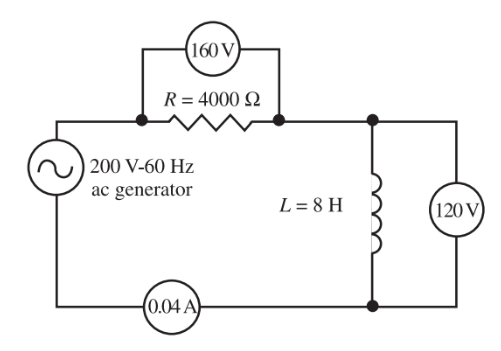Figure 9. A circuit having an 8-Henry choke, 4000-ohm resistor connected across a 200-volt, 60 Hz ac source.

First, find the reactance of L.

${{X}_{L}}=2\pi fL=2\pi *60Hz*8H=3014\Omega$

Find the impedance of the circuit.

$Z=\sqrt{{{R}^{2}}+X_{L}^{2}}=\sqrt{{{4000}^{2}}+{{3000}^{2}}}=5000\Omega$

Find the current in the circuit.

$I=\frac{E}{Z}=\frac{200V}{5000\Omega }=0.04A$

Find the voltage drop across R and XL.

\begin{align} & {{E}_{R}}=I*R=0.04A*4000\Omega =160V \\ & {{E}_{{{X}_{L}}}}=I*{{X}_{L}}=0.04A*3000\Omega =120V \\\end{align}

Notice that the sum of the voltage drops does not equal the applied voltage. This is because the two voltages are 90 degrees out of phase. This requires vector addition.

$Source\text{ }Voltage={{E}_{S}}=\sqrt{{{160}^{2}}+{{120}^{2}}}=200V$

Find the phase angle θ between I and E.

\begin{align} & \cos \theta =\frac{R}{Z}=\frac{4000\Omega }{5000\Omega }=0.8 \\ & \theta =co{{s}^{-1}}\left( 0.8 \right)={{37}^{o}} \\\end{align}

Find the true power and apparent power.

The apparent power equals:

$Apparent\text{ }Power=E\times I=200\times 0.04A=8VA$

The true power equals:

$True\text{ }Power=E\times I\times \cos \theta =200V\times 0.04A\times .8=6.4W$

Note that some figures have been approximated to make the calculations simpler.

## Parallel RL Circuit

In a circuit containing a resistance and an inductance in parallel, the voltage of each circuit element is the same as the source voltage. Further, there is no phase difference between the elements because they are all in parallel.

There will, however, be a phase difference among the total and branch currents. The current is in phase with voltage in the resistive branch. Current lags the voltage across the inductor by 90 degrees.

• The total current will lag the source voltage by some angle between 0 and 90 degrees.
• If the reactance of the inductor is larger than the resistance of the resistor, the angle will be closer to 0 degrees.
• If the resistance is much larger than the reactance the lag will be closer to 90 degrees.

In the parallel RL circuit, we do not find impedance through a vector sum. Instead, we apply Ohm’s law.

$Z=\frac{{{E}_{S}}}{{{I}_{T}}}$

As discussed, the voltage in the parallel RL circuit is in phase with IR, and it leads IL by 90 degrees. Thus, we can say that IR leads IL by 90 degrees.

The parallel RL circuit, then, has two current components—IR and IL. Both of these can be represented by phasors. Since they are out of phase, we cannot simply add the two components together to figure the total circuit current. We must find their phasor sum. See Figure 10.

${{I}_{T}}=\sqrt{I_{R}^{2}+I_{L}^{2}}$

Note that the phasor for IL is below the horizontal reference. This is because IL lags voltage—the horizontal reference for the parallel RL circuit. (Since a phasor, or rotating vector, rotates counterclockwise, a lagging phasor would be behind, or clockwise too, a leading phasor.)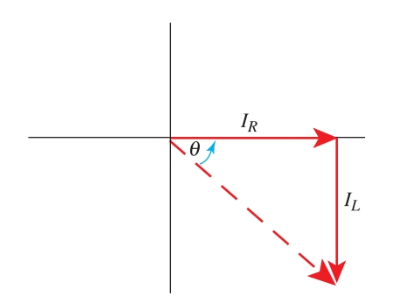Figure 10. Phasor diagram showing θ relation with IR and IC.

## Phase Angle Definition

The phase angle represents the phase displacement between current and voltage resulting from a reactive circuit element.

For the parallel RL circuit, the phase angle is found on the current phasor diagram. The horizontal reference of this circuit is voltage since it is common to all circuit elements.

In the current phasor diagram, we do not have a voltage phasor. We use IR as the horizontal component since it is in phase with voltage, Figure 10. Using this diagram, the phase angle can be found from:

$\theta =\arctan \left( \frac{{{I}_{L}}}{{{I}_{R}}} \right)$

## Impedance Calculation Example

Using the circuit with the assigned values in Figure 11, determine the phase angle between applied voltage and current. Draw the current phasor diagram and find the circuit impedance.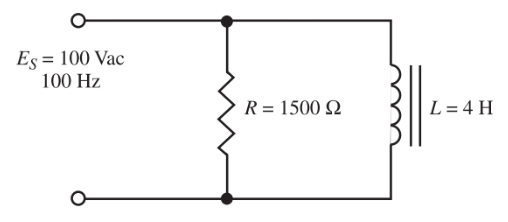Figure 11. A parallel RL circuit.

Step 1. Compute the value of inductive reactance, XL.

${{X}_{L}}=2\pi fL=2\pi *100Hz*4H=2512\Omega$

Step 2. Compute the branch currents. Using Ohm’s law:

\begin{align} & {{I}_{R}}=\frac{{{E}_{S}}}{R}=\frac{100V}{1500\Omega }=0.067A \\ & {{I}_{L}}=\frac{{{E}_{S}}}{{{X}_{L}}}=\frac{100V}{2512\Omega }=0.04A \\\end{align}

Step 3. Determine the phase angle to see how much the circuit current lags the voltage.

$\theta =\arctan \left( \frac{{{I}_{L}}}{{{I}_{R}}} \right)=\arctan \left( \frac{0.04}{0.067} \right)={{30.8}^{o}}$

Step 4. Draw the current phasor diagram. Use any convenient scale. Remember that IR is drawn as the horizontal component. IL is drawn downward at 90 degrees from IR since it lags voltage—the horizontal reference. See Figure 12.Figure 12. Current phasor diagram.

Step 5. Find the total circuit current.

${{I}_{T}}=\sqrt{I_{R}^{2}+I_{L}^{2}}=\sqrt{{{0.067}^{2}}+{{0.04}^{2}}}=0.078A$

Step 6. Find the impedance of the circuit. Using Ohm’s law:

$Z=\frac{{{E}_{S}}}{{{I}_{T}}}=\frac{100V}{0.078A}=1282\Omega$

Once again, it is important to understand how the various quantities would be affected by a change in frequency. The effects of changing frequency are summarized in Figure 13. It shows how values change in a parallel RL circuit as the frequency is changed and inductance is held at a constant value.Figure 13. This table shows the effect on various values in a parallel RL circuit as the frequency is changed and the inductance value is held constant.

Did you find apk for android? You can find new Free Android Games and apps.